# GSEB Solutions Class 11 Chemistry Chapter 6 Thermodynamics

Gujarat Board GSEB Textbook Solutions Class 11 Chemistry Chapter 6 Thermodynamics Textbook Questions and Answers.

## Gujarat Board Textbook Solutions Class 11 Chemistry Chapter 6 Thermodynamics

### GSEB Class 11 Chemistry Thermodynamics Text Book Questions and AnswersQuestion 1.
Choose the correct answer. A thermodynamic state function is a quantity
(i) used to determine heat changes
(ii) whose value is independent of path
(iii) used to determine pressure volume work
(iv) whose value depends on temperature only.
(ii) State function does not depend upon the path followed or is independent of the path followed.

Question 2.
For the process to occur under adiabatic conditions, the correct condition is :
(i) ∆T = 0
(ii) ∆p = 0
(iii) q = 0
(iv) w = 0
(iii) No heat is allowed to enter or leave the system under adiabatic conditions.

Question 3.
The enthalpies of all elements in their standard states are:
(i) unity
(ii) zero
(iii) < 0
(iv) different for each element
(ii) The enthalpies of all elements in their standard states are zero.Question 4.
∆UΘ of combustion of methane is – χ kJ mol-1. The value of ∆UΘ is
(i) = ∆UΘ
(ii) > ∆UΘ
(iii) < ∆UΘ
(iv) = 0
(iii) ∆UΘ = ∆UΘ – 2RT as ∆n = – 2.

Question 5.
The enthalpy of combustion of methane, graphite and dihydrogen at 298 K are – 890.3 kJ mol-1 – 393.5 kJ mol-1, and – 285.8 kJ mol-1 respectively. Enthalpy of formation of CH4(g) will be:
(i) – 74.8 kJ mol-1
(ii) – 52.27 kJ mol-1
(iii) + 74.8 kJ mol-1
(iv) + 52.26 kJ mol-1.
Given:
(a) CH4(g) + O2(g) → CO2(g) + 2H2O(1); ∆H = – 890.3 kJ mol-1
(b) C(graphite) + O2(g) → CO2(g); ∆H = – 393.5 kJ mol-1
(c) H2(g) +$$\frac { 1 }{ 2 }$$O2(g) → H2O(l); ∆H = – 285 kJ mol-1
Our aim to get the enthalpy of formation of CH4(g),
i. e., C(graphite) + 2H2(g) → CH4(g); ∆H = ?
C(graphite) + 2H2(g) + O2(g) – CH4(g) – O2(g) → CO2(g) +
2H2O(l) – CO2(g) – 2H2O(1); ∆H = – 393.5 + 2(- 285.8) – (- 890.3) kJ mol-1
C(graphite) + 2H2(g) → CH4(g)
∆H = – 393.5 – 571.6+ 890.3 kJ mol-1
= – 74.8 kJ mol-1

Question 6.
A reaction, A + B → C + D + q is found to have a positive entropy change. The reaction will be –
(i) possible at high temperature
(ii) possible only at low temperature
(iii) not possible at any temperature
(iv) possible at any temperature
The given reaction is
A + B → C + D + q
or A + B → C + D; AH = – q
i. e. The reaction is exothermic
∆G = AH – T∆S
∆S for the reaction is given to be positive
∴ The reaction is spontaneous or possible if ∆G is -ve now ∆H is already -ve
∴ The reaction is possible at any temperature or (iv) Choice is correct.Question 7.
In a process, 701 J of heat is absorbed by a system and 394 J of work is done by the system. What is the change in internal energy for the process?
∆U = q + w; where ∆U is change of internal energy.
Given q = 701 J
Since the work is done by the system; w is -ve
∆U = q – w = (701 – 394) J = 307 J
∴ Increase in internal energy = 307 J.

Question 8.
The reaction of cyanamide, NH2CN(s), with dioxygen was,carried out in a bomb calorimeter, and ∆U was found to be – 742.7 kJ mol-1 at 298 K. Calculate enthalpy change for the reactibn at 298 K.
NH2CN(S) + $$\frac { 3 }{ 2 }$$O2(g) → N2(g) + CO2(g) + H2O(l)
Solution:
The given reaction is
NH2CN(s) + $$\frac { 3 }{ 2 }$$O2(g) → N2(g) + CO2(g) + H2O(l)
∆U = – 742.7 kJ mol-1
Enthalpy change ∆H = AU + ∆ngRT
where ∆ngg = change in the no. of moles (gaseous)
= n2 – n1
= 2 – $$\frac { 3 }{ 2 }$$ = $$\frac { 1 }{ 2 }$$
∆H = – 742.7 + $$\frac { 1 }{ 2 }$$(0.083)(298)
= – 741.5 kJ
∴ Enthalpy change for the reaction is – 741.5 kJ.

Question 9.
Calculate the number of kJ of heat necessary to raise the temperature of 60.0 g of aluminium from 35°C to 55°C. Molar heat capacity of Al is 24 J mol-11 K-1
Solution:
Mass of Al given = 60.0 g
∆T = rise in temp = (55 – 35) = 20.0°C
No. of moles of Al = $$\frac { 60.0 }{ 27 }$$
Molar heat capacity of Al = 24 K mol-1 K-1
∴ q = Total heat required= n x C x ∆T
= $$\frac { 60.0 }{ 27 }$$ x 20.0 x 24.0 J = 1066.7 J
= 1.07 kJ

Question 10.
Calculate the enthalpy change of freezing of 1.0 mol of water at 10.0°C to ice at – 10.0°C. ∆fus = 6.03 kJ mol-11 at 0°C.
Solution:
(i) Heat change required to lower the temperature of water from 10.0°C to 0°C .
∆H1 = n x CP x ∆T = 1.0 x 75.3 x (- 10) = – 753 J mol-1

(ii) Heat change required to convert 1 mol. of H2O(l) at 0°C to H2O at 0°C
∆H2 = ∆Hfusion = – 6.03 kJ mol-1 as heat is given out

(iii) Heat change reqd to change 1 mole of ice from 0°C to -10.0°C
∆H3 = – 36.8 x 10 x 1 = – 368 J mol-1
Total heat change = ∆H1 + ∆H2 + ∆H3
= (- 0.753 – 6.03- 0.368) kJ mol-1
∴ Total enthalpy change = – 7.151 kJ mol-1
As in each step, heat is evolved, each step will have a negative sign with ∆H.Question 11.
Enthalpy of combustion of carbon to CO2 is – 393.5 kJ mol-1. Calculate the heat released upon formation of 35.2 g of CO2 from carbon and dioxygen gas.
Solution: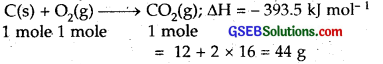For formation of 44.0 g of CO2, heat released is = – 393.5 kJ
For formation of 35.2 g of CO2, heat released is
= $$\frac { -393.5 }{ 44 }$$ x 35.2 = – 314.8 kJ
∴ Heat released = – 314.8 kJ or – 315 kJ

Question 12.
Enthalpies of formation of CO(g), CO2(g), N2O(g) and N2O4(g) are – 110, – 393, 81 and 9.7 kJ mol-1 respectively. Find the value of ∆H for the reaction:
N2O4(g) + 3CO(g) → N2O(g) + 3CO2(g)
Solution:
fH(CO) = – 110 kJ mol-1
fH(CO2) = – 393 kJ mol-1
fH(N2O) = 81.0 kJ mol-1
fH(N2O4) = 9.7 kJ mol-1
The given reaction is
N2O4(g) + 3CO(g) → N2O(g) + 3CO2(g); ∆rH = ?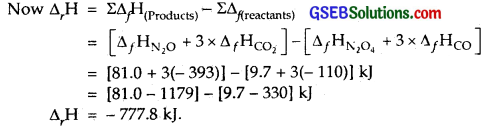Question 13.
Given
N2(g) + 3H2(g) → 2NH3(g); ∆rHΘ = – 92.4 kJ mol-1 What is the standard enthalpy of formation of NH3 gas?
Solution:
Given : N2(g) + 3H2(g) → 2NH3(g); ∆rHΘ = – 92.4 kJ mol-1
This is the heat evolved for 2 moles of NH3(g)
∴Heat evolved for 1 mole of NH3(g) = $$\frac { -92.4 }{ 2 }$$ = – 46.2 kJ
Hence ∆rHΘ of NH3 gas = – 46.2 kJ mol-1

Question 14.
Calculate the standard enthalpy of formation of CH3 OH(l) from the following data :
CH3OH(l) + $$\frac { 3 }{ 2 }$$O2(g) → CO2(g) + 2H2O(l); ∆rHΘ = – 726 kJ mol-1
C(graphite) + O2(g) → CO2(g); ∆cHΘ= – 393 kJ mol-1
H2(g) + $$\frac { 1 }{ 2 }$$O2(g) → H2O(l); ∆fHΘ = – 286 kJ mol-1
Solution:
Given data is:
(i) CH4OH(l) + $$\frac { 3 }{ 2 }$$O2(g) → CO2(g) + 2H2O(l); ∆rHΘ = – 726 kJ mol-1

(ii) C(s) + O2(g) → CO2(g); ∆cHΘ = – 393 kJ mol-1

(iii) H2(g) + $$\frac { 1 }{ 2 }$$ O2(g) → H2O(l); ∆fHΘ = – 286 kJ mol-1
Our aim is to get
C(s) + 2H2(g) + 2O2(g) – CH3OH(l) – $$\frac { 3 }{ 2 }$$O2(g) → CO2(g) + 2H2O(l) – CO2(g) – 2H2O(l)
or C(s) + 2H2(g) + $$\frac { 1 }{ 2 }$$O2(g) → CH3OH(l); ∆fHΘ [- 393 + 2(- 286) – (- 726)]
= – 239 kJ mol-1
Thus the enthalpy of formation of CH3OH(l) = – 239 kJ mol-1.Question 15.
Calculate the enthalpy change for the process CCl4(g) → C(g) + 4Cl(g) and calculate bond enthalpy change of C-Cl in CCl4(g)
vapHΘ(CCl4) = 30.5 kJ mol-1,
fHΘ(CCl4) = – 135.5 kJ mol-1
aHΘ(C) = 715.0 kJ mol-1 where ∆aHΘ is enthalpy of atomisation
aHΘ(C) = 242 kJ mol-1.
Solution:
Here we are given
(i) CCl4(g) → C(g) + 4Cl(g); ∆vapHΘ(CCl4) = 30.5 kJ mol-1

(ii) C(s) + 2Cl2 = CCl4(1); ∆fHΘ(CCl4) = – 135.5 kJ mol-1

(iii) C(s) → C(g); ∆gHΘ(C) = 715.0 kJ mol-1
where ∆aHΘ is the enthalpy of atomisation

(iv) Cl2(g) → 2Cl(g); ∆aHΘ(Cl2) = 242 kJ mol-1
Out aim is CCl4(g) → C(g) + 4(Cl(g); ∆rHΘ = ?
Add (i) and (ii), subtract (iii) and (iv) x 2
CCl4(l) + C(s) + 2Cl2(g) – C(s) – 2Cl2(g) → CCl4(g) + CCl4(l) – C(g) – 4Cl(g)
or ∆rHΘ = 30.5 – 135.5 – 715 – 484 = – 1304 kJ
0 = CCl4(g) – C(g) + 4 Cl(g); ∆rHΘ = – 1304 kJ
or CCl4(g) → C(g) + 4Cl(g); ∆rHΘ = 1304 kJ
There are 4 bonds of C – Cl in CCL4
∴ Bond enthalpy, of C – Cl bond = $$\frac { 1304 }{ 4 }$$ = 326 kJ mol-1

Question 16.
For an isolated system, ∆U = 0, what will be ∆S?
Solution: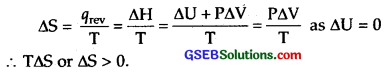Question 17.
For the reaction at 298 K,
2A + B → C
∆H = 400 kJ mol-1 and ∆S = 0.2 kJ K-1 mol-1.
At what temperature will the reaction become spontaneous considering ∆H and ∆S to be constant over the temperature range.
Solution:
For the reaction 2A + B → C;
∆H = 400 kJ K-1 mol-1 and ∆S = 0.2 kJ K-1 mol-1
∆G = ∆H-T∆S
For a spontaneous reaction ∆G has to be negative
∆H is 400 kJ mol-1 (positive)
∴ T∆S has to be > ∆H
or T > $$\frac { ∆H }{ ∆S }$$ > $$\frac { 400 }{ 0.2 }$$
or T > 2000 K
Thus for the reaction to be spontaneous, temperature should be > 2000 K.

Question 18.
For the reaction,
2Cl(g) → Cl2 → (g), what are the signs of ∆H and ∆S?
Solution:
2Cl(g) → Cl2(g) indicates the formation of bonds between the two chlorine atoms to form Cl2 molecule.
During bond formation energy is released.
∴ ∆H is negative
Randomness decreases as Cl atoms combine to form Cl2.
∴ ∆S is also negative                   [ ∵ There is less randomness in molecules than in atoms ]Question 19.
For the reaction 2A(g) + B(g) → 2D(g)
∆UΘ = – 10.5 kj and ∆SΘ = – 44.1 JK-1
Calculate ∆GΘ for the reaction, and predict whether the reaction may occur spontaneously.
Solution:
For the given reaction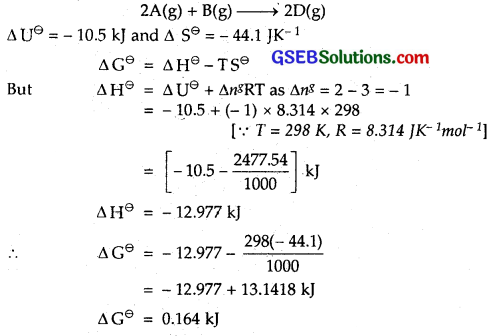Since ∆GΘ is positive
∴ reaction is not spontaneous.

Question 20.
The equilibrium constant for a reaction is 10. What will be the value of ∆GΘ? R = 8.314 JK-1 mol-1 , T = 300 K.
Solution:
The equilibrium given for a reaction is 10
i.e. K = 10
∆G = – 2.303 RT log K
= – 2.303 x 8.314 x 300 log 10 J
= – 2.303 x 8.314 x 300 x 1                   [As log 10 = 1]
= – 5744.42 J
= – 5.744 kJ mol-1.Question 21.
Comment on the thermodynamic stability of NO(g), given
$$\frac { 1 }{ 2 }$$ N2(g) + $$\frac { 1 }{ 2 }$$O2(g) → NO(g); ∆rHΘ = 90 kJ mol-1
NO(g) + $$\frac { 1 }{ 2 }$$O2(g) → NO2(g); ∆rHΘ = – 74 kJ mol-1
Solution:
$$\frac { 1 }{ 2 }$$ N2(g) + $$\frac { 1 }{ 2 }$$ O2(g) → NO(g); ∆rHΘ = 90 kJ mol-1
NO(g) + $$\frac { 1 }{ 2 }$$O2(g) → NO2(g); ∆rHΘ = – 74 kJ mol-1
As is evident from the above, NO(g) is unstable but NO2(g) is formed since energy is absorbed in the first case and it is released in the second case.

Question 22.
Calculate the entropy change is surrounding when 1.00 mol of H2O(l) is formed under standard conditions. ∆fHΘ = – 286 kJ mol-1.
Solution:
H2(g) + O2→ H2O(l); ∆HΘ = – 286 kJ mol-1
Since heat (enthalpy) is evolved in the above reaction.
∴ It is absorbed by the surroundings .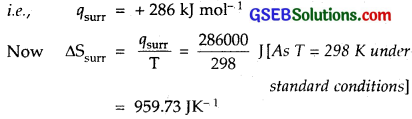∴ Entropy change in surroundings = 959.73 JK-1 mol-1